﻿ Finite Element Analysis for the Modelling of Building Structures in Three Dimensional Schemes

### Finite Element Analysis for the Modelling of Building Structures in Three Dimensional Schemes

Czeslaw Miedzialowski, Joanna KretowskaOPEN ACCESSPEER-REVIEWED

## Finite Element Analysis for the Modelling of Building Structures in Three Dimensional Schemes

Czeslaw Miedzialowski1, Joanna Kretowska2,1Facultyof Civil and Environmental Engineering, Bialystok University of Technology, Bialystok, Poland

2PopeJohn II State School ofHigher Education, BialaPodlaska, Poland

### Abstract

Three-dimensional analysis of building structure is a very complex problem and solution of this problem is often obtained via finite element method.The paper presents a set of finite elements used for the modelling of building structures taking into account soil-structures interaction. Finite elements of the structure are derived by using beam schemes including Timoshenko type beam. The finite elements descriptions are completed by plate state. The way of elements connections and the global system of equations are also defined. The finite elements of wall panels, floor slabs, joints ant contact type subsoilproposed in this study significantly reduce the number of unknowns. Two computational examples proved the efficiency and the computing possibilities of the presented model.

### At a glance: Figures

1234
Prev Next

• Miedzialowski, Czeslaw, and Joanna Kretowska. "Finite Element Analysis for the Modelling of Building Structures in Three Dimensional Schemes." American Journal of Civil Engineering and Architecture 2.4 (2014): 143-148.
• Miedzialowski, C. , & Kretowska, J. (2014). Finite Element Analysis for the Modelling of Building Structures in Three Dimensional Schemes. American Journal of Civil Engineering and Architecture, 2(4), 143-148.
• Miedzialowski, Czeslaw, and Joanna Kretowska. "Finite Element Analysis for the Modelling of Building Structures in Three Dimensional Schemes." American Journal of Civil Engineering and Architecture 2, no. 4 (2014): 143-148.

 Import into BibTeX Import into EndNote Import into RefMan Import into RefWorks

### 1. Introduction

A suitable analysis of building structures which describes internal forces redistribution requires three dimensional static schemes which take into consideration soil-structure interaction. The buildings elements such as wall panels and floor slabs should be analysed taking into account its shield and plate work which is important from the point of view of the existing loads: elements dead load, floor slab useful load, wall wind load, seismic and paraseismic effects, pressure caused by gas explosion, no uniform settlement etc.

Three-dimensional description of building structure is a very complex problem and solution of this problem is often obtained by using finite element method. The computational model, which uses classical finite elements, incorporates substantial disadvantages as a result of high time-consuming computations (high number of degrees of freedom) and extended data processing.

In practice the real modelling of building structures in three-dimensional schemes requires using special algorithms and elements. The computational method presented in [5, 6] describes plane elements in which the theory of Timoshenko-type beam has been used to define wall and floor elements but the compression and twisting have been added to the classical formulation. This method shows that the efficient 3D analysis of building structures which provide accurate results with significantly reduced number of unknowns (in comparison to the classic FEM) can be done.

Many building structures are constructed using coupled shear walls or shear wall-frame systems. Therefore, much research on efficient analysis of such structures has been undertaken. Many authors present methods which employ super elements [1, 2, 3, 8]. Plane stress elements and beam elements have been used to model the shear wall core and frames. In  super elements are developed using the matrix condensation technique. Fictitious beams are used in the development of super element. Shear walls may have openings for functional reason. The location, number and size of openings affects the structure behaviour. Kim et al. in  present a seismic analytical model for the analysis of high-rise buildings structure with shear walls and openings. In  authors develop a closed-form super element method for tall buildings of irregular geometry. The building is divided into a very small number of super elements and for each the stiffness matrix and load vector are derived.

This paper deals with a three dimensional semi-analytical model of building structure which allows for the natural division into constituting elements (wall and floor slabs, joints). The elements description (including finite element work description taking into account plate state) is presented in the paper.

### 2. The Computational Model

2.1. Description of Wall and Floor Elements

The finite elements of wall panels, floor slabs, joints, and subsoil are necessary for the description of 3D structures taking into consideration soil-structure interaction, Figure 1.

The loads in elements plane in quasi two-dimensional finite elements are reduced to the nodes loads and carried by shield state of load-bearing strip elements. In fact, the loads are applied to the element surface which causes element work also in plate state.

In the presented model wall elements are defined with respect to the shield state but floor slabs elements with respect to the shield state and plate state (Figure 2.).

Shield state is described by the model of Thimoshenko-type beam  but the compression and twisting have been added to the classical formulation Figure 2.a. Element geometry is determined by the height of the storey and width of the bay of the building.

The displacement field of floor elements is expressed by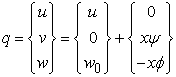(1)

where: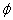- angle of rotation of the strip cross-section,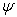- angle of twist of the strip cross-section.

The strain field is written as: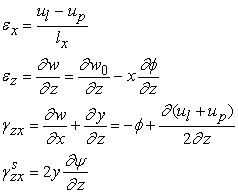(2)

Strain ez is composed of the part due to uniformly distributed compression ezw and the part resulting from bending ezf. The strain vector has therefore the following form(3)

where:

l, p - two adjacent points between which strain is

averaged,

lx - distance between points l and p (width of the strip).

L- differential operator matrix,(4)

The stress field is determined as follows:(5)

where:

D –constitutive matrix of shield-type elements.(6)

So the stiffness matrix K quasi two-dimensional element with respect to the shield state can be defined by: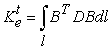(7)

In order to enable easier implementation of connections of finite elements, according to the presented model, the unknown displacements are transferred to the corners of elements.

Hence, vector of unknown displacements qt has the form: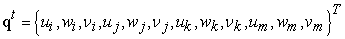(8)

Plate state has been defined by substitute beam system which consists of four elements shown in Figure 2b.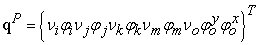(9)

Stiffness matrix can be obtained from the potential energy balance: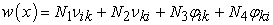(10)

where displacement state is approximated as follows:(11)

Shape functions have a form::(12)

The stiffness matrix can be defined from the general relationship (Eq.7).

Stiffness matrix of element in plate state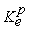is obtained by aggregation of stiffness matrix components of individual beam elements.

Load vector of element in plate state can be expressed by: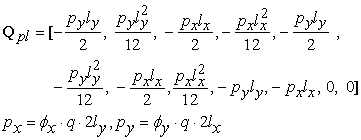(13)

where:

q – intensity of surface load,

px – substitute beam load in x direction,

py- substitute beam load in y direction,

fx, fy – load distribution coefficients according to  in x and y direction,

lx, ly – half length of side of element in x and y direction.

Taking into account shield state and plate state the finite element presented in Figure 3. has been obtained.

2.2. Joint Description

In  8-node three-dimensional joint element has been used between wall elements, Figure 4a. The walls elements are modelled as semi-analytical shear elements. The distribution of the unknown displacements in 8-node spatial joint is shown in Figure 4b. Displacement field and strain field ez is assumed as in the three-dimensional state of stress.

Strain field assumed as in the three-dimensional state has a form: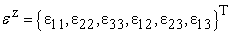(14)(15)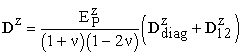(16)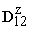– matrix 6x6 in which: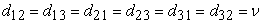and the other elements are equal to 0.

Figure 4. Three-dimensional joint model a) interconnections between 8-node spatial joint element and wall elements, b) 8-node spatial joint element

Vector of unknown displacements(Figure 3a) has the form(17)

The distribution of the unknown displacements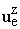can be described by(18)

Shape function is defined by transforming the linear function at corner nodes to the nodes assumed in strip planes in the following form: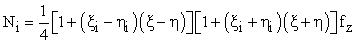(19)

fz – component of the distribution in the direction of z-axis which has the linear form.

Graph of N3 shape function is shown in Figure 5.

2.3. Subsoil Model

The subsoil model of the approximated elastic half-space which allows to determine vertical displacements presented by Switka in  has been used. This mechanical subsoil model contact type is presented in Figure 6.

The forces of subsoil interaction have the form: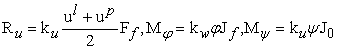(20)

where

Ff– cross-sectional area of the foundation footing,

Jf– moment of inertia of the foundation footing,

Jo – polar moment of inertia of the foundation footing,

ku, kv, kw – proportional subsoil coefficients.

Soil-structure interaction system is shown in Figure 7.

Taking into account the soil-structure interaction, the computational model has a form: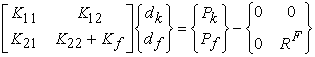(21)

where: k – degree of freedom of the structure,

f – degree of freedom of the contact zone,

between structure and subsoil,

RF - forces of subsoil interaction.

The aggregation of elements of a three-dimensional building structure shown in Figure 1. has to be done in global coordinate system according to formula :(22)(23)

where

re - constituting elements (wall panels, floor slabs, joints),

e – one type elements.

Taking into consideration boundary conditions the final matrix system of equations which describes three-dimensional work of structure has a form: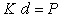(24)

where:

K – global stiffness matrix of the structure,

d - unknown vector,

### 3. Numerical Verification

3.1. Element in the Plate State

The element work with respect to plate state, has been tested by using stiffness matrix described in the presented beam model. The results have been compared to the results obtained by using classic plate finite elements. To minimize accuracy error,a number of elements 30x30 have been used. The analyses have been carried out for two slabs (one with simply supported edges and second with fixed edges) showed in Figure 8.(the ratio of edges length from 1:1 to 2:1). The basic slab span was 3 m. and thickness 15 cm. The C20/25 concrete has been used. Unit load has been applied. The bending moments in the middle of the slab have been compared and presented in Table 1. and Table 2.

#### Table 2. Computational results – beam model

3.2. Interactive Three Dimensional System

The apartment building erected in 1978/1979 in Bialystok (Poland). This is a 5-storey building structure with basement, built of pre-fabricated (large-panel building technology). The building was investigated in the process of development and during the first year of using . Building structure had prefabricated panel walls and floors (thickness 14 cm) and solid concrete strips footings. The height of the storey is 2.7 m. There are sandy subsoil and clayey subsoil under the building. The dead weight and useful load are taken into consideration. The object of this test is to compare the settlements of building structure determined in-situ and obtained by using described model. Figure 9. shows the horizontal projection and the measuring points location. The finite elements of prefabricated elements size have been used in the computational model presented in the paper.

The results of building structures settlements are presented in Figure 10. and in Table 3.

### 4. Conclusions

A set of finite elements for the analysis of 3D building structures taking into account the plate state of elements and soil-structure interaction has been presented.

The substitute beam systems which allow to analyze bi-directional bending slabs have been described. The test has been carried out for two slabs with the ratio of edges length from 1:1 to 2:1 - Figure 8. The substitute beam system has been loaded according to the load distribution factors presented in .

The computational results shown in Table 1 proved that the substitute beam system could be useful for the modelling of bi-directional bending slabs to obtain the internal forces and that the method is very effective in comparison to the similar plate system analyzed by using classical FEM, because of much lower number of unknowns.

The 3D large panel system building has been analysed taking into consideration soil-structure interactions.

The settlements of building structure obtained by using the described model provided accurate results in comparison to the settlements determined in-situ (Table 2.).

The model which takes into account plate state of wall elements is more rigid but the results remain comparable to those obtained in-situ.

The building structure model is assembled of elements corresponding to the division of a real structure into constituent elements (wall panels, floor slabs and joints) so the discretization does not cause any difficulties.

### References

  H.S. Kim, D.G. Lee, “Analysis of shear wall with openings using super elements,” Engineering Structures, 25. 981-991. 2003.In article CrossRef  H.S. Kim, D.G. Lee, “Efficient analysis of flat slab structures subjected to lateral loads,” Engineering Structures, 27. 251-263. 2005.In article CrossRef  H.S. Kim, D.G. Lee, Ch. K. Kim, “Efficient three-dimensional seismic analysis of a high-rise building structure with shear walls,” Engineering Structures, 27. 963-976.2005In article  Kobiak J., Stachurski W., Reinforced concrete structutes, Arkady, Warsaw, 1984.In article  MiedziałowskiCz, “Three dimensional modelling wall structures for buildings,” Archives of Civil Engineering, XLI (2), 195-212. 1995.In article  MiedzialowskiCz. “Modelling of subsoil flexibility in computations of complex three-dimensional structural schemes of buildings,” Archives of Civil Engineering, XLII, (1), 83-102.1996.In article  Steckiewicz R., Basewicz E., Romanczuk E., Monitoring in the stage of realization of large-panel building OWT, Design and Research Works Department, Miastoprojekt, Bialystok, 1980 (in polish).In article  SteenbergenR.D.J.M., BlaauwendraadJ., “Closed-form super element method for tall buildings of irregular geometry”, International Journal of Solids and Structures, 44. 5576-5597. 2007.In article CrossRef  Switka R., Approximated elastic half-space for the modelling elastic subsoil, Poznan Technical University, Poznan, 1968. (in polish).In article  ThimoshenkoS.P.., GoodierJ.N., Theory of elasticity, McGraw-Hill, New York, 1951In article  Zienkiewicz O. C., Taylor R. L. The finite element method, Butterworth-Heinemann, 2005.In article